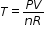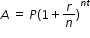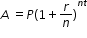# MA.912.AR.1.2Export Print
Rearrange equations or formulas to isolate a quantity of interest.

### Examples

Algebra 1 Example: The Ideal Gas Law PV = nRT can be rearranged asto isolate temperature as the quantity of interest.

Example: Given the Compound Interest formula, solve for P

Mathematics for Data and Financial Literacy Honors Example: Given the Compound Interest formula, solve for t.

### Clarifications

Clarification 1: Instruction includes using formulas for temperature, perimeter, area and volume; using equations for linear (standard, slope-intercept and point-slope forms) and quadratic (standard, factored and vertex forms) functions.

Clarification 2: Within the Mathematics for Data and Financial Literacy course, problem types focus on money and business.

General Information
Subject Area: Mathematics (B.E.S.T.)
Strand: Algebraic Reasoning
Status: State Board Approved

## Related Courses

This benchmark is part of these courses.
1200310: Algebra 1 (Specifically in versions: 2014 - 2015, 2015 - 2022, 2022 and beyond (current))
1200320: Algebra 1 Honors (Specifically in versions: 2014 - 2015, 2015 - 2022, 2022 and beyond (current))
1200370: Algebra 1-A (Specifically in versions: 2014 - 2015, 2015 - 2022, 2022 and beyond (current))
1200380: Algebra 1-B (Specifically in versions: 2014 - 2015, 2015 - 2022, 2022 and beyond (current))
1200400: Foundational Skills in Mathematics 9-12 (Specifically in versions: 2014 - 2015, 2015 - 2022, 2022 and beyond (current))
7912080: Access Algebra 1A (Specifically in versions: 2014 - 2015, 2015 - 2018, 2018 - 2019, 2019 - 2022, 2022 and beyond (current))
7912090: Access Algebra 1B (Specifically in versions: 2014 - 2015, 2015 - 2018, 2018 - 2019, 2019 - 2022, 2022 and beyond (current))
1200315: Algebra 1 for Credit Recovery (Specifically in versions: 2014 - 2015, 2015 - 2022, 2022 and beyond (current))
1200375: Algebra 1-A for Credit Recovery (Specifically in versions: 2014 - 2015, 2015 - 2022, 2022 and beyond (current))
1200385: Algebra 1-B for Credit Recovery (Specifically in versions: 2014 - 2015, 2015 - 2022, 2022 and beyond (current))
7912075: Access Algebra 1 (Specifically in versions: 2014 - 2015, 2015 - 2018, 2018 - 2019, 2019 - 2022, 2022 and beyond (current))
1210305: Mathematics for College Statistics (Specifically in versions: 2022 and beyond (current))
1200388: Mathematics for Data and Financial Literacy Honors (Specifically in versions: 2022 and beyond (current))
1200384: Mathematics for Data and Financial Literacy (Specifically in versions: 2022 and beyond (current))
7912120: Access Mathematics for Data and Financial Literacy (Specifically in versions: 2022 - 2023 (current), 2023 and beyond)
1200710: Mathematics for College Algebra (Specifically in versions: 2022 and beyond (current))

## Related Access Points

Alternate version of this benchmark for students with significant cognitive disabilities.
MA.912.AR.1.AP.2: Rearrange an equation or a formula for a specific variable.

## Related Resources

Vetted resources educators can use to teach the concepts and skills in this benchmark.

## Formative Assessments

Solving Formulas for a Variable:

Students are given the slope formula and the slope-intercept equation and are asked to solve for specific variables.

Type: Formative Assessment

Solving Literal Equations:

Students are given three literal equations, each involving three variables and either addition or subtraction, and are asked to solve each equation for a specific variable.

Type: Formative Assessment

Literal Equations:

Students are given three literal equations, each involving three variables and either multiplication or division, and are asked to solve each equation for a specific variable.

Type: Formative Assessment

Solving a Literal Linear Equation:

Students are given a literal linear equation and asked to solve for a specific variable.

Type: Formative Assessment

Surface Area of a Cube:

Students are asked to solve the formula for the surface area of a cube for e, the length of an edge of the cube.

Type: Formative Assessment

Rewriting Equations:

Students are given a literal equation involving four variables and are asked to solve for the variable in the quadratic term.

Type: Formative Assessment

## Lesson Plans

Free Fall Clock and Reaction Time!:

This will be a lesson designed to introduce students to the concept of 9.81 m/s2 as a sort of clock that can be used for solving all kinematics equations where a = g.

Type: Lesson Plan

Efficient Storage:

The topic of this MEA is work and power. Students will be assigned the task of hiring employees to complete a given task. In order to make a decision as to which candidates to hire, the students initially must calculate the required work. The power each potential employee is capable of, the days they are available to work, the percentage of work-shifts they have missed over the past 12 months, and the hourly pay rate each worker commands will be provided to assist in the decision process. Full- and/or part-time positions are available. Through data analysis, the students will need to evaluate which factors are most significant in the hiring process. For instance, some groups may prioritize speed of work, while others prioritize cost or availability/dependability.

Type: Lesson Plan

Graphing vs. Substitution. Which would you choose?:

Students will solve multiple systems of equations using two methods: graphing and substitution. This will help students to make a connection between the two methods and realize that they will indeed get the same solution graphically and algebraically.  Students will compare the two methods and think about ways to decide which method to use for a particular problem. This lesson connects prior instruction on solving systems of equations graphically with using algebraic methods to solve systems of equations.

Type: Lesson Plan

## Perspectives Video: Professional/Enthusiast

Have a need for speed? Get out your spreadsheet! Race car drivers use algebraic formulas and spreadsheets to optimize car performance.

Type: Perspectives Video: Professional/Enthusiast

## Tutorial

Solving a literal equation:

Students will learn to solve a literal equation.

Type: Tutorial

## Video/Audio/Animation

Solving Literal Equations:

Literal equations are formulas for calculating the value of one unknown quantity from one or more known quantities. Variables in the formula are replaced by the actual or 'literal' values corresponding to a specific instance of the relationship.

Type: Video/Audio/Animation

## STEM Lessons - Model Eliciting Activity

Efficient Storage:

The topic of this MEA is work and power. Students will be assigned the task of hiring employees to complete a given task. In order to make a decision as to which candidates to hire, the students initially must calculate the required work. The power each potential employee is capable of, the days they are available to work, the percentage of work-shifts they have missed over the past 12 months, and the hourly pay rate each worker commands will be provided to assist in the decision process. Full- and/or part-time positions are available. Through data analysis, the students will need to evaluate which factors are most significant in the hiring process. For instance, some groups may prioritize speed of work, while others prioritize cost or availability/dependability.

## MFAS Formative Assessments

Literal Equations:

Students are given three literal equations, each involving three variables and either multiplication or division, and are asked to solve each equation for a specific variable.

Rewriting Equations:

Students are given a literal equation involving four variables and are asked to solve for the variable in the quadratic term.

Solving a Literal Linear Equation:

Students are given a literal linear equation and asked to solve for a specific variable.

Solving Formulas for a Variable:

Students are given the slope formula and the slope-intercept equation and are asked to solve for specific variables.

Solving Literal Equations:

Students are given three literal equations, each involving three variables and either addition or subtraction, and are asked to solve each equation for a specific variable.

Surface Area of a Cube:

Students are asked to solve the formula for the surface area of a cube for e, the length of an edge of the cube.

## Student Resources

Vetted resources students can use to learn the concepts and skills in this benchmark.

## Tutorial

Solving a literal equation:

Students will learn to solve a literal equation.

Type: Tutorial

## Video/Audio/Animation

Solving Literal Equations:

Literal equations are formulas for calculating the value of one unknown quantity from one or more known quantities. Variables in the formula are replaced by the actual or 'literal' values corresponding to a specific instance of the relationship.

Type: Video/Audio/Animation

## Parent Resources

Vetted resources caregivers can use to help students learn the concepts and skills in this benchmark.